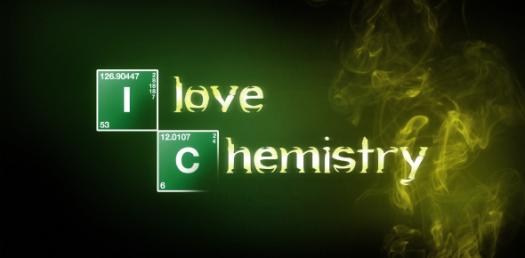# Chemistry Practice Test Questions! Trivia Quiz

13 Questions | Total Attempts: 469SettingsAre you looking for some chemistry practice test questions? One of the ways that you can ensure you have a good understanding of a subject is to ensure that you understand the basics. In this trivia quiz, we seek to test one's knowledge of basic chemistry, how about you try it out and check out other quizzes like it for practice.

• 1.
All isotopes of tin have the same I. number of protons.              II. number of neutrons.           III. mass number
• A.

I only

• B.

II only

• C.

III only

• D.

D. I and III only I and III only

• 2.
Which of the following particles contain more electrons than neutrons? I.          11H                  II         3417Cl-                     III        3919K+
• A.

I only

• B.

II only

• C.

I and II only

• D.

II and III only

• 3.
Isotopes of an element differ in their:
• A.

Atomic number

• B.

Position in the Periodic Table

• C.

Number of neutrons

• D.

Electronic arrangement

• 4.
Isotopes of an element have the same number of
• A.

Protons and electrons

• B.

Neutrons and electrons

• C.

Protons and neutrons

• D.

Protons, neutrons and electrons.

• 5.
Which species have electronic configurations 2.8.8, 2.8 and 2.8.1 respectively?
• A.

Ne, F, Na

• B.

K+, F- , Mg2+

• C.

Ca2+ , F, Na+

• D.

Cl- , F- , Na

• 6.
What information about the structure of a hydrogen atom can be gained from its emission spectrum?
• A.

Most of the mass of the atom is in its nucleus

• B.

A hydrogen atom contains one proton and one electron.

• C.

The electron in the hydrogen atom is held near the nucleus.

• D.

The electron may exist in any of several energy levels.

• 7.
Spectra have been used to study the arrangements of electrons in atoms. An emission spectrum consists of a series of bright lines that converge at high frequencies. Such emission spectra provide evidence that electrons are moving from
• A.

Lower to higher energy levels with the higher energy levels being closer together.

• B.

Lower to higher energy levels with the lower energy levels being closer together.

• C.

Higher to lower energy levels with the lower energy levels being closer together.

• D.

Higher to lower energy levels with the higher energy levels being closer together.

• 8.
Consider the composition of the particles W, X, Y, Z below. Which two particles are isotopes of the same element?   Particle Number of protons Number of neutrons Number of electrons W 11 12 10 X 12 12 12 Y 12 13 12 Z 13 14 10
• A.

W and X

• B.

X and Y

• C.

Y and Z

• D.

W and Z

• 9.
What is the electron configuration of an atom of element 20?
• A.

8.8.4

• B.

4.8.8

• C.

2.8.10

• D.

2.8.8.2

• 10.
Isotopes of an element have the same
• A.

Number of protons and neutrons

• B.

Number of protons and electrons

• C.

Atomic number and mass number number of neutrons and electrons

• D.

Atomic number and mass number

• 11.
Which electron transition in the hydrogen atom releases the most energy?
• A.

N=2→ n = 1

• B.

N=3→ n = 2

• C.

N=4→ n = 3

• D.

N=5→ n = 4

• 12.
Isotopes are elements with
• A.

The same atomic number and the same number of neutrons.

• B.

The same mass number but a different number of neutrons

• C.

The same atomic number but a different number of neutrons.

• D.

Different atomic and mass numbers but the same number of neutrons

• 13.
Which two species contain the same number of neutrons?
• A.

55Mn and 56Fe

• B.

35Cl and 37Cl

• C.

23Na and 39K

• D.

32S and 35Cl

Related TopicsBack to top